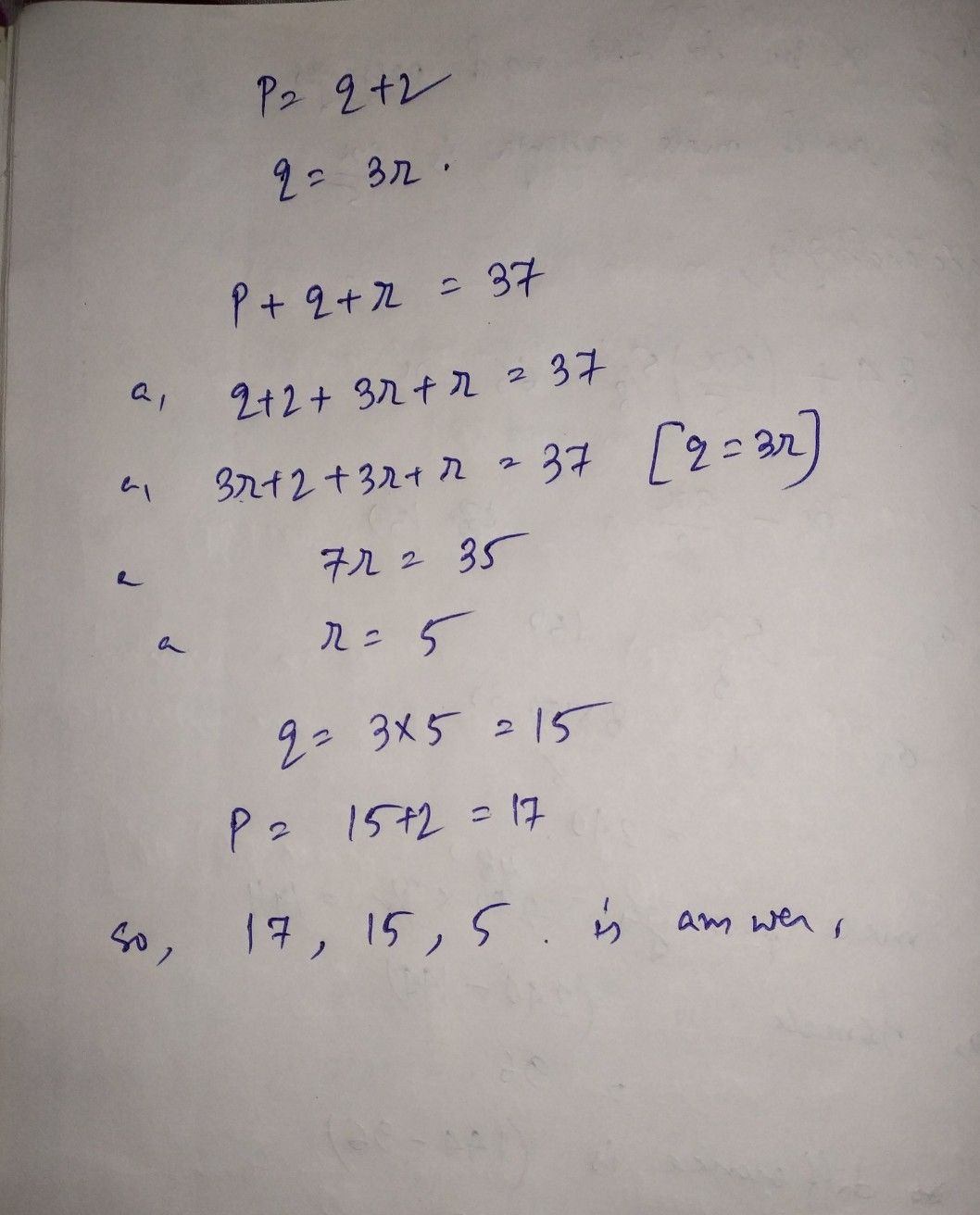Symbol
ProblemIn a trangle, suppose that the length of a side p is two mches longer than side g. The length of side gis three times 25 long as side $+8\pi D505z$ that the perimeter of the triangle $15$ $3=$ mches. Find the length of each side. $\left(z$ $-$ $17=$ $25$ $2=5=$ (b) $18=2\pi$ $155\pi 5$ $=$ $5\pi$ $E\right)$ $1=$ $15^{-}$ $1-$ $d\right)$ $17=$ $15$ n.5 $\bar{I} ^{x}\pi$
Other
SolutionQanda teacher - shubapaul
option d is correct